New update is available. Click here to update.

# Split BST

Last Updated: 28 Mar, 2021
Difficulty: Moderate

## PROBLEM STATEMENT

#### Note:

``````1. A binary search tree is a binary tree data structure, with the following properties
a. The left subtree of any node contains nodes with the value less than the node’s value.
b. The right subtree of any node contains nodes with the value equal to or greater than the node’s value.
c. Right, and left subtrees are also binary search trees.
2. It is guaranteed that all nodes in the given tree will have distinct positive integral values .
3. The given tree may or may not contain a node of value equal to the given value ‘K’.
``````

#### Example, below the tree, is a binary search tree.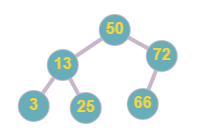#### Below the tree is not a BST as node ‘2’ is less than node ‘3’ but ‘2’ is the right child of ‘3’, and node ‘6’ is greater than node ‘5’ but it is in the left subtree of node ‘5’.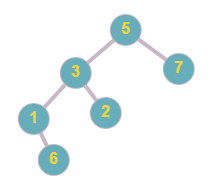#### Note:

``````1. You have to split the given tree in such a way that the structure of both returned trees is similar to the originally given tree, i.e. if a parent node ‘P’ and child node ‘C’ lies on the same tree after splitting, then ‘C must be the same child of ‘P’.
2. If there is no valid tree, then return ‘NULL’ node in its place.
``````

#### Input Format:

``````The first line contains an integer 'T' which denotes the number of test cases or queries to be run.

The first line of each test case contains elements of the tree in the level order form. The line consists of values of nodes separated by a single space. In case a node is null, we take -1 in its place.

The second line of each test case contains a positive integer value ‘K’, denoting the value for splitting the given tree.

For example, the level order input for the tree depicted in the below image.
````````````50
13 72
3 25 66 -1
-1 -1 -1 -1 -1 -1
``````

#### Explanation :

``````Level 1 :
The root node of the tree is 50

Level 2 :
Left child of 50 = 13
Right child of 50 = 72

Level 3 :
Left child of 13 = 3
Right child of 13 = 25
Left child of 72 = 66
Right child of 72 =  ‘Null’

Level 4 :
All children are ‘Null’

The first not-null node(of the previous level) is treated as the parent of the first two nodes of the current level. The second not-null node (of the previous level) is treated as the parent node for the next two nodes of the current level and so on.
The input ends when all nodes at the last level are null(-1).
``````

#### Note :

``````The above format was just to provide clarity on how the input is formed for a given tree.
The sequence will be put together in a single line separated by a single space. Hence, for the above-depicted tree, the input will be given as:
50 13 72 3 25 66 -1 -1 -1 -1 -1 -1 -1
``````

#### Output Format:

``````For each test case, the output will be “1” if you have returned the correct answer, else it will be “0”.
The output of each test case will be printed in a separate line.
``````

#### Note :

``````You do not need to input or print anything, and it has already been taken care of. Just implement the given function.
``````

#### Constraints:

``````1 <= T <= 5
1 <= N <= 3000
1 <= data <= 10 ^ 9
1 <= K <= 10 ^ 9
Where ‘T’ denotes the number of test cases, ‘N’ is the total number of nodes in the given binary tree, ‘data’ is the value of the nodes of the given binary tree, and ‘K’ represents the given value for splitting the tree.

For a single test case, all given ‘data’ are distinct from each other.
``````

#### Sample Input 1:

``````2
7 5 10 3 6 8 11 2 -1 -1 -1 -1 -1 -1 -1 -1 -1
5
5 4 6 -1 -1 -1 -1
3
``````

#### Sample Output 1:

``````1
1
``````

#### Explanation of Sample Input 1:

``````For the first test case, the given tree is.
``````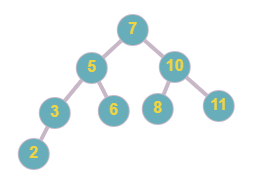``````All nodes less than or equal to ‘5’ will be part of the first tree and all remaining nodes will be part of the second tree. The tree after the split will look like the one below.
``````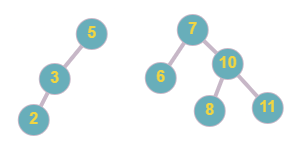``````The given tree in the second test case is -
``````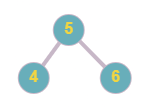``````All nodes in the tree are greater than the given value, thus we will return ‘NULL’ node in the first tree and the original tree as the second tree.
``````

#### Sample Input 2:

``````1
10 2 12 1 3 -1 13 -1 -1 -1 -1 -1 -1
11
``````

#### Sample Output 2:

``````1
``````

#### Explanation of Sample Input 2:

``````For the first test case, the given tree is
``````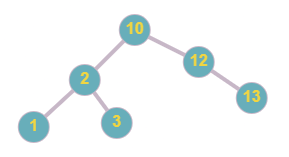``````The output trees are below.
``````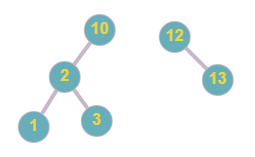``````Note that the below output tree is not correct because the structure is not maintained as node ‘12’ and ‘13’ lies on the same tree and ‘12’ is a child of ‘13’ in the output tree, but in the original tree ‘13’ is a child of ‘12’.
``````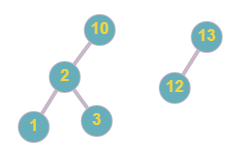## Approach 1

As we know that in a binary search tree every node in the left subtree has value less than the value of the root node and every node in the right subtree has value greater than the value of the root node. Thus if the root node’s value is less than or equal to the given value ‘K’ then after splitting the tree, this root node must be included in the ‘first’ tree, and also we can assure that the left subtree of this root node will also be part of ‘first’ tree, as all nodes of left subtree must have a value less then ‘K’, Or if the root node’s value is greater than the given value ‘K’, then this root node will be included in the ‘second’ tree and the right subtree of the root node will also be included in the ‘second’ tree as all nodes in right subtree must have a value greater than ‘K’. So the idea here is to check the root node, if its value is less than or equal to ‘K’, then include the whole left subtree with the root node in the ‘first’ tree. But there may be some nodes in the right subtree that can have a value less than ‘K’, so recursively call the function to check for the right child of the root node. If the root node’s value is greater than ‘K’ include a whole right subtree with the root node and recursively call the function to check the left child of the root node.

## Algorithm:

• Declare two node variable say ‘first’ and ‘second’ where ‘first’ is the root node of a tree having nodes with values smaller or equal than given value ‘K’, and ‘second’ is the root node of a tree having nodes with values greater than ‘K’.
• Call ‘splitTree’ function with the given ‘root’ node of the original tree and store returned trees in first and second.
• Return [ ‘first’, ‘second’ ]

### Description of ‘splitTree’ function

The function will take two parameters:

• ‘root’: root node of the tree that is to be split.
• ‘K’: integer value for splitting the given tree.

TreeNode[] splitTree ( ‘root’, ‘k’ ):

• Base case, If ‘root’ is NULL, then return two NULL nodes.
• If the value of the root is less than or equal to the given value.
• Recursively call the ‘split’ function with ‘root -> right’ and store returned trees in variables say ‘retFirst’ and ‘retSecond’.
• Set ‘first’ to ‘root’ and change ‘first -> right’ to ‘retFirst’.
• Set ‘second’ to ‘retSecond’.
• Else
• Recursively call the ‘split’ function with ‘root -> left’ and store returned trees in variables say ‘retFirst’ and ‘retSecond’.
• Set ‘second’ to ‘root’ and change ‘second -> left’ to ‘retSecond’.
• Set ‘first’ to ‘retFirst’.
• Return two tree nodes - [ ‘first’, ‘second’ ].